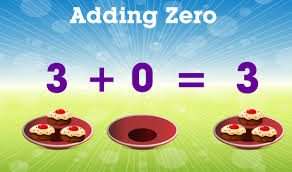# ZERO CONCEPT-P.1

##### This unit is about the use of zero by adding,subtracting and multiplying by zero

Zero concept:

Adding zero to a number

Any number plus zero is that number.

Examples:

5+ 0=5

8+ 0 =8

12 + 0=12

20 + 0=20

Exercise

4 + 0 =

15 + 0 =

7 + 0 =

30 + 0 =

Add more numbers for practice.

Adding a number to zero

Zero plus any number is that number.

Examples:

0 + 2 = 2

0 + 6 = 6

0 + 10 = 10

0 + 24 = 24

Add more numbers for practice.

Subtracting zero from a number.

Any number minus /take away zero is that number.

Examples:

6 – 0 = 6

12 – 0 = 12

28 – 0 = 28

Add more numbers for practice.

Multiplying by zero

Any number times 0 is 0 ( 0 groups of any number is 0)

0 x 2 = 0

3 x 0 = 0

0 x 8 = 0

Exercise

Multiply

0 x 5 =         0 x 10 =       0 x 12 =     15 x 0 =

0 x 1 =         9 x 0 =         0 x 7 =       0 x 13 =

0 x 20 =       63 x 0 =       4 x 0 =       5 x 0 =

Add more numbers for practice.

SEE ALL
•YOU